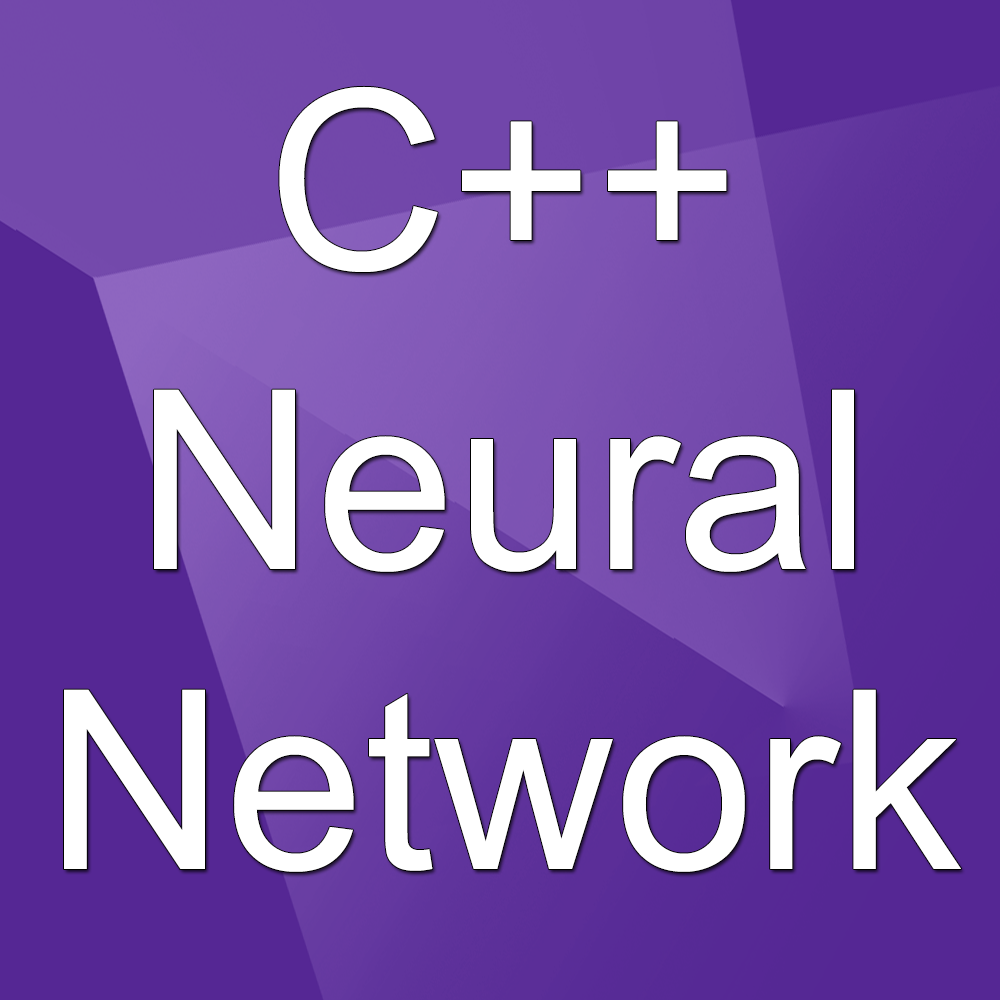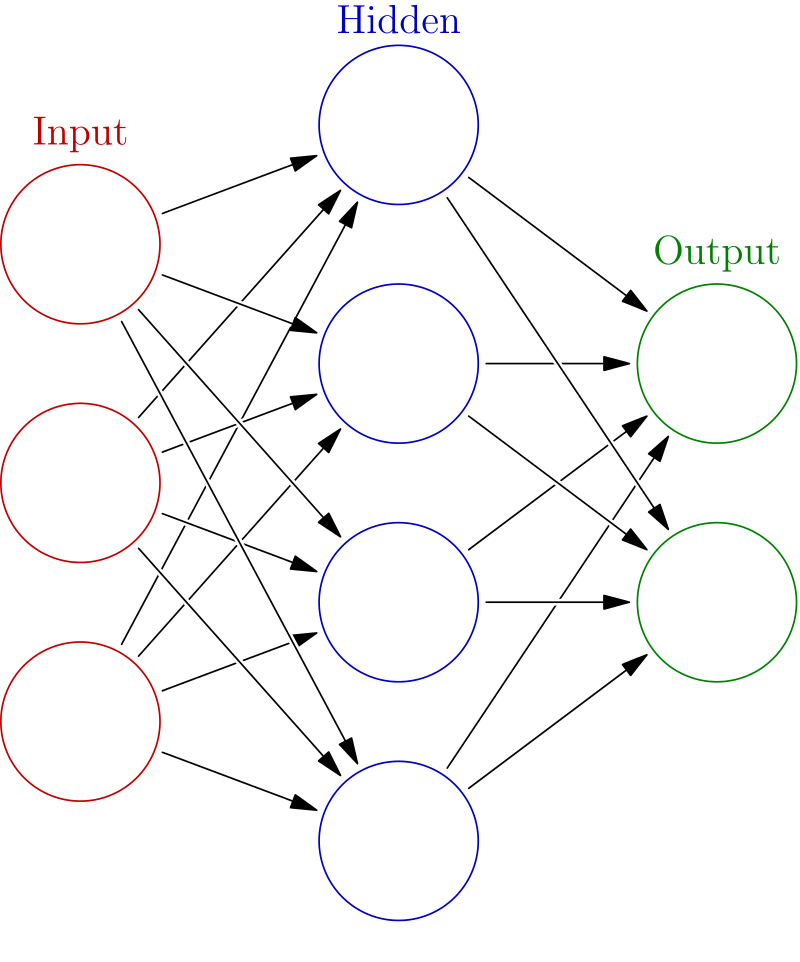# Neural Network in C++ From Scratch and Backprop-Free OptimizersIn this article I’ll present a beginner-oriented framework implementing neural networks in C++. The main goal of this code is to understand the root of neural networks for beginners, it also allows multiple modifications which are usually hard to implement in classical high-level framework (Pytorch, Tensorflow, Keras etc..).

## Introduction

In this article I’ll present a beginner-oriented framework implementing neural networks in C++. The main goal of this code is to understand the root of neural networks for beginners, it also allows multiple modifications which are usually hard to implement in classical high-level framework (Pytorch, Tensorflow, Keras etc..).

So this code can also be used for some research because you can also do things you couldn’t do in other frameworks.

1. Features of the framework
2. Neural networks from scratch
3. Framework design
4. Exotic backpropagation-free optimizer: Shaking Tree optimizer
5. What I learned doing this
6. Github repository

Let’s go!

## 1. Features of the framework

• Graph implementation of neural network (no matrices)
• Neural network, neurons and edges as explicit objects
• Only multilayer perceptrons with Linear/ReLU/Sigmoid activations
• No parallel computations, no optimizations, no convolutional layers
• Exotic backpropagation-free optimizer

## 2. Neural networks from scratch

Neural networks can be interpreted in two ways. The “graph” way and the “matrix” way. When you begin with neural networks, people usually teach you the “graph” way:## Different Optimization Algorithm for Deep Neural Networks: Complete Guide

In this article, we will only focus on the Better Optimizing algorithm for Deep Neural Network (DNN). We will call this optimizing algorithm as a Learning algorithm for this article.

## Alibaba’s Mobile Neural Network: A deep learning framework for mobile and embedded devices

A peek at Alibaba’s Mobile Neural Network (MNN) and how it achieves balance between high performance, flexibility, and ease-of-use.

## Deep Learning 101 —  Neural Networks Explained

The past few decades have witnessed a massive boom in the penetration as well as the power of computation, and amidst this information.

## Explain Deep Learning Neural Networks to your grandma

“You do not really understand something unless you can explain it to your grandmother” Not sure where this quote originally came from, it is sometimes kind of half-attributed to Albert Einstein.

## Deep Learning Explained in Layman's Terms

Deep Learning Explained in Layman's Terms. In this post, you will get to learn deep learning through a simple explanation (layman terms) and examples.